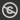Science, Maths & Technology
Free course

# Univariate continuous distribution theory## Course reviews

This free course looks at a number of the basic properties of statistical models. Section 1 is concerned with the distributions of continuous random variables which are described by their probability density functions (pdfs) and cumulative distribution functions (cdfs). Section 2 is concerned with moments and covers, the concept of expectation or expected value, the familiar notion of the mean, also known as the first moment, two general definitions of moments, variance, random variables linked by linear transformation and finally, how to deal with moments all in one go, using the moment generating function.

## Course learning outcomes

After studying this course, you should be able to:

• understand the properties of probability density functions and cumulative distribution functions
• define expectation, and be introduced to its important linearity property
• calculate raw moments and central moments, including their special cases, the mean and variance
• understand the effect of linear transformation on mean, variance and density
• calculate the moment generating function, and appreciate its link to moments.

First Published: 12/03/2018

Updated: 10/06/2019

Skip Rate and Review